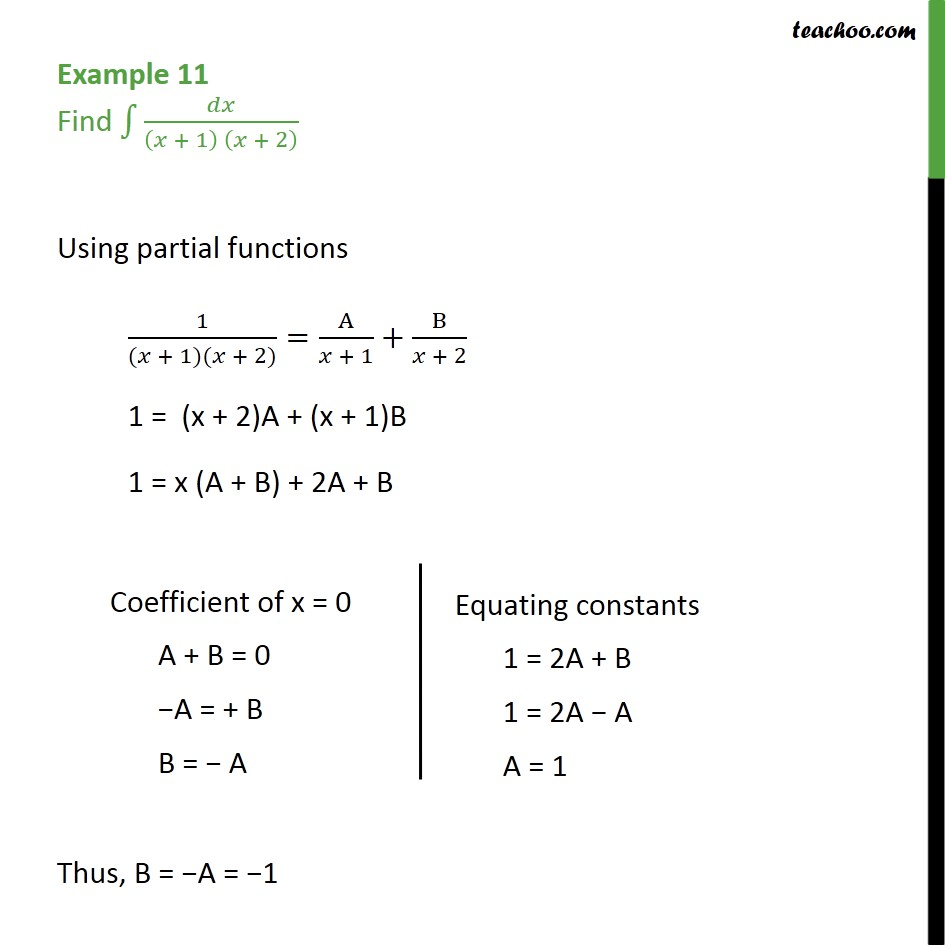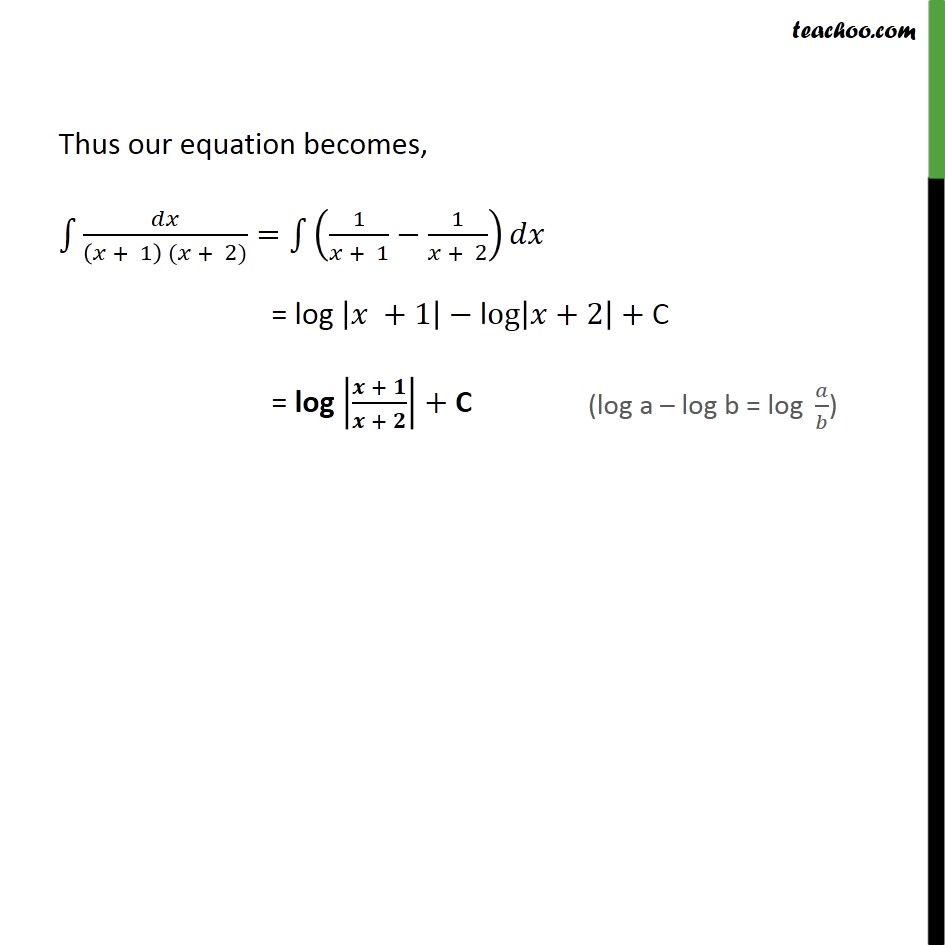Integration by partial fraction - Type 1

Chapter 7 Class 12 Integrals
Concept wiseLearn in your speed, with individual attention - Teachoo Maths 1-on-1 Class

### Transcript

Example 11 Find + 1 + 2 Using partial functions 1 ( + 1)( + 2) = A + 1 + B + 2 1 = (x + 2)A + (x + 1)B 1 = x (A + B) + 2A + B Thus, B = A = 1 Thus our equation becomes, + 1 ( + 2) = 1 + 1 1 + 2 = log +1 log +2 + C = log + + + C